Courses

# Exercise Problem - Flow of Ideal Fluids Mechanical Engineering Notes | EduRev

## Mechanical Engineering : Exercise Problem - Flow of Ideal Fluids Mechanical Engineering Notes | EduRev

The document Exercise Problem - Flow of Ideal Fluids Mechanical Engineering Notes | EduRev is a part of Mechanical Engineering category.
All you need of Mechanical Engineering at this link: Mechanical Engineering

Exercise Problems  -  Chapter 7

1. Determine whether the function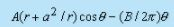is a possible velocity potential for inviscid incompressible flow.

2. Find the stream function and the velocity potential for flow around a closed contour formed by superposition of a source, sink and a rectilinear flow. The source and sink are of equal strength and they are located at equal distances on either side of the origin along the x-axis.

3. Verify the stream function and the velocity potential for flow around a circular cylinder with Circulation satisfying their respective Laplace equations.

4. A kite is having cross-sectional area of .5 m ×.5 m. The weight of kite is 4.9 N and it flies in the air at an angle of 100 with the horizontal plane. The string of the kite makes an angle of 450 with the horizontal plane. The tension applied by the person flying the kite is N. If the velocity of the wind at the layer where the kite is flying is 15 m/s, find out the lift and drag coefficients of the kite.

Take ρ of air as 1.2 kg/m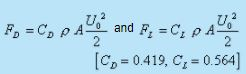5. In a tornado (free vortex motion), at a radius of 20 cm the velocity (tangential component is the only non-trivial component) and pressures are 22.5 m/s and 117.72 kpa absolute. Find out the pressure at a radius of 4.5 m. The fluid is air and assumed to have density equal to 1.2 kg/m3 .

[196.705 kpa]

6. Flow past a rotating cylinder can be simulated by superposition of a doublet, a uniform flow and a vortex. The peripheral velocity of the rotating cylinder alone is given by ν0 at r = R (R is the radius of the cylinder). Use the expression for the combined velocity potential for the superimposed uniform flow, doublet and vortex flow (clockwise rotation) and show that the resultant velocity at any point on the cylinder is given by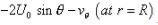. The angle θ is the angular position of the point of interest. A cylinder rotates at 600 rpm around its own axis which is perpendicular to the uniform air stream density 1.24 kg/m3 having a velocity of 40 m/s. The cylinder is 2 m in diameter. Find out (a) circulation,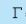(b) lift per unit length and the (c) position of the stagnation points.

[394.78 m2 / s, 19581.29 N/m, -51.750 and 231.750 ]

7. In the early attempts for the development of the aeroplane, rotating cylinders were used as the airfoils. Consider such a cylinder having a diameter of 1m and a length of 10 m. If this cylinder is rotated at 120 rpm while the plane moves at a speed of 120 km/hour through the air at 700 m standard atmosphere ( ρ = 0.59 kg/m3 ), estimate the minimum lift that could be developed disregarding end effects.

[ 3880 N]

8. If a cylinder (placed with its axis normal to the free stream ) is to be used as a pitot-static tube for the measurement of the free-stream velocity, find the locations of piezo-metric holes on the cylinder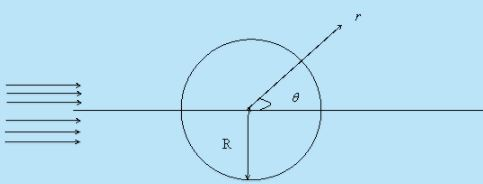Assume ideal fluid flow. The velocity components in the flow field are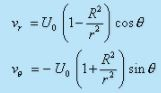Note: A Pitot-static tube measures the velocity head, i.e., the difference between the stagnation pressure and static pressure in the flow.

[1800, 2100]

Recap

In this course you have learnt the following

• Irrotationality leads to the condition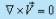which demands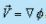, where φ is known as a potential function. For a potential flow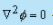.
• The stream function also obeys the Laplace’s equation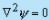for the potential flows. Laplace’s equation is linear, hence any number of particular solutions of Laplace’ s equation added together will yield another solution. So a complicated flow for an in viscid, incompressible, irrotational condition can be synthesized by adding together a number of elementary flows which are also inviscid, incompressible and irrotational. This is called the method of superposition.
• Some inviscid flow configurations of practical importance are solved by using the method of superposition. The circulation in a flow field is defined as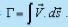. Subsequently , the velocity may be defined as circulation per unit area . The circulation for a closed path in an irrotational flow field is zero. However , the circulation for a given closed path is an irrotational flow containinga finite number of singular points is a non -zero constant.
• The lift around an immersed body is generated when the flow field processes circulation. The lift around a body of any shape is given by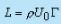, where ρ is the density and U0 is the velocity in the streamwise direction.
Offer running on EduRev: Apply code STAYHOME200 to get INR 200 off on our premium plan EduRev Infinity!

,

,

,

,

,

,

,

,

,

,

,

,

,

,

,

,

,

,

,

,

,

;